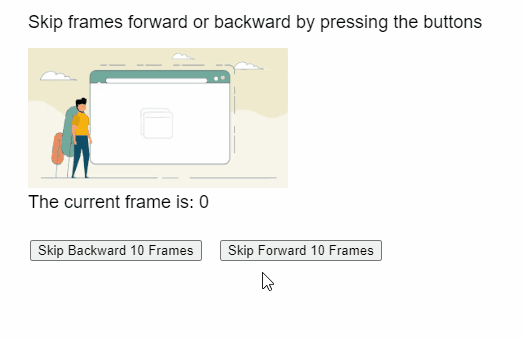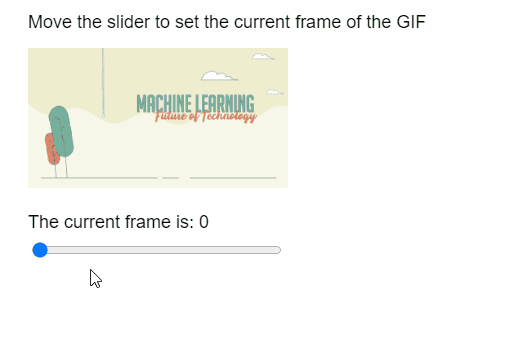# p5.js Image setFrame() Method

The setFrame() method of p5.Image in p5.js library is used to set the index of the currently visible frame of the GIF animation.

Syntax:

```setFrame( index )
```

Parameters: This function accepts a single parameter as mentioned above and described below.

• index: It is a number that denotes the index of the frame to be displayed.

The following libraries are included in the “head” section of the HTML page while implementing the following examples.

<script src=”p5.Image.js”></script>
<script src=”p5.min.js”></script>

Example 1: The examples below illustrates the setFrame() method in p5.js library.

## Javascript

 `function` `preload() { ` `  ``example_gif = ` `    ``loadImage(``"sample-gif.gif"``); ` `} ` ` `  `function` `setup() { ` `  ``createCanvas(500, 300); ` `  ``textSize(18); ` ` `  `  ``example_gif.pause(); ` ` `  `  ``decFrameBtn = ` `    ``createButton(``"Skip Backward 10 Frames"``); ` `  ``decFrameBtn.position(30, 240); ` `  ``decFrameBtn.mousePressed(skipBackward); ` ` `  `  ``incFrameBtn = ` `    ``createButton(``"Skip Forward 10 Frames"``); ` `  ``incFrameBtn.position(220, 240); ` `  ``incFrameBtn.mousePressed(skipForward); ` `} ` ` `  `function` `draw() { ` `  ``clear(); ` ` `  `  ``text(``"Skip frames forward or backward "` `+ ` `       ``"by pressing the buttons"``, 20, 20); ` ` `  `  ``// Draw the GIF on screen ` `  ``image(example_gif, 20, 40, 260, 140); ` ` `  `  ``// Get the current frame ` `  ``let currFrame = ` `      ``example_gif.getCurrentFrame(); ` ` `  `  ``text(``"The current frame is: "` `+ ` `       ``currFrame, 20, 200); ` `} ` ` `  `function` `skipForward() { ` ` `  `  ``// Get the current playing frame of the GIF ` `  ``let currFrame = ` `      ``example_gif.getCurrentFrame(); ` ` `  `  ``// Move forward only if possible ` `  ``if` `(currFrame < ` `      ``example_gif.numFrames() - 10) { ` `       `  `    ``// Add 10 to skip forward ` `    ``let newFrame = currFrame + 10; ` ` `  `    ``example_gif.setFrame(newFrame); ` `  ``} ` `} ` ` `  `function` `skipBackward() { ` ` `  `  ``// Get the current playing frame of the GIF ` `  ``let currFrame = ` `      ``example_gif.getCurrentFrame(); ` ` `  `  ``// Move forward only if possible ` `  ``if` `(currFrame > 10) { ` `       `  `    ``// Subtract 10 to skip forward ` `    ``let newFrame = currFrame - 10; ` ` `  `    ``example_gif.setFrame(newFrame); ` `  ``} ` `}`

Output:Example 2:

## Javascript

 `function` `preload() { ` `  ``example_gif = ` `    ``loadImage(``"sample-gif.gif"``); ` `} ` ` `  `function` `setup() { ` `  ``createCanvas(500, 300); ` `  ``textSize(18); ` ` `  `  ``example_gif.pause(); ` ` `  `  ``let totalFrames = ` `      ``example_gif.numFrames() - 1; ` ` `  `  ``frameSlider =  ` `    ``createSlider(0, totalFrames, 0, 1); ` `  ``frameSlider.position(30, 240); ` `  ``frameSlider.size(250); ` `} ` ` `  `function` `draw() { ` `  ``clear(); ` ` `  `  ``text(``"Move the slider to set the "` `+ ` `       ``"current frame of the GIF"``, 20, 20); ` ` `  `  ``// Draw the GIF on screen ` `  ``image(example_gif, 20, 40, 260, 140); ` ` `  `  ``// Set the current frame according to the ` `  ``// value of the slider ` `  ``example_gif.setFrame(frameSlider.value()); ` ` `  `  ``// Get the current frame ` `  ``let currFrame = ` `      ``example_gif.getCurrentFrame(); ` ` `  `  ``text(``"The current frame is: "` `+ ` `       ``currFrame, 20, 220); ` `} `

Output:Online editor: https://editor.p5js.org/
Environment Setup: https://www.geeksforgeeks.org/p5-js-soundfile-object-installation-and-methods/
Reference: https://p5js.org/reference/#/p5.Image/setFrameMy Personal Notes arrow_drop_upIm listening

If you like GeeksforGeeks and would like to contribute, you can also write an article using contribute.geeksforgeeks.org or mail your article to contribute@geeksforgeeks.org. See your article appearing on the GeeksforGeeks main page and help other Geeks.

Please Improve this article if you find anything incorrect by clicking on the "Improve Article" button below.

Article Tags :

Be the First to upvote.

Please write to us at contribute@geeksforgeeks.org to report any issue with the above content.